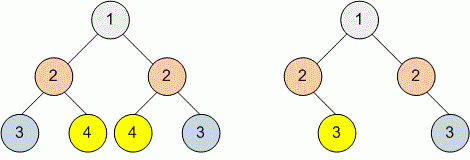Competitions

# Tree Symmetric

Given a binary tree. Check whether it is a mirror of itself (i.e. symmetric around its center).

Definition of a tree:

// Java
class TreeNode
{
public:
int val;
TreeNode left;
TreeNode right;
TreeNode(int x) {
val = x;
left = NULL;
right = NULL;
};

// C++
class TreeNode
{
public:
int val;
TreeNode *left;
TreeNode *right;
TreeNode(int x) : val(x), left(NULL), right(NULL) {}
};


Implement function isSymmetric that returns true if tree is symmetric and false otherwise.

// Java
boolean isSymmetric(TreeNode tree)

// C++
bool isSymmetric(TreeNode *tree)


#### ExampleFunction isSymmetric returns true for the first tree and false for the second one.

Time limit 1 second
Memory limit 128 MiB
Author Mykhailo Medvediev